# Warping

My research is essentially an attempt to extend the overall framework of general relativity to include other basic forces or “fundamental interactions”. This page summarises that framework and covers some related notions. As the name suggests, it discusses the warping of spacetime. The page “breaking” discusses notions of symmetry and its breaking, leading up to a summary of my recent research.

Both pages are intended to be accessible to the intelligent layperson. However, I’m also aware that many readers may be researchers in the field themselves, while some will have studied physics for several years. As a result, I’ve kept these pages fairly concise, to ensure that a) I don’t bore the more experienced readers too much and b) that I don’t have to spend years writing them! I therefore rattle through the concepts at quite a pace, which I hope doesn’t leave the lay readers too dizzy. I’m sure those reading these pages will be able to judge for themselves whether it’s time to take a break and make a cup of coffee/tea/hot chocolate (or whatever beverage you feel like) and mull over what they’ve read. I hope that in this way, I’m striking a decent balance that’s ok for all levels of experience.

Rather than launch straight in to general relativity, I thought it helpful to start with some basic physics concepts which set the theory in context.

## Newtonian Mechanics

A basic question in physics is this. Say you’ve got a moving object. What causes it to change its motion, and how does this motion change? Before the twin revolutions of relativity and quantum theory rocked physics in the early 20th Century, this was the subject of a branch of physics called mechanics. The rules of mechanics were set out by Sir Isaac Newton in 1687.Newton said that the velocity of an object only changes if it is acted on by a force. He then described one such force mathematically. He said that the same force, gravity, causes a ball to trace a curved path when it flies through the air and the planets to revolve around the sun.

## Vectors and scalars

An equation that describes the change of an object’s motion is, sensibly enough, called an “equation of motion”. Equations of motion often include quantities known as vectors. These are quantities which have a direction as well as a size.

For example, the Newtonian equation of motion relates the force applied to an object to the object’s resulting acceleration. Force and acceleration are both vectors: I can apply a certain amount of force to an object in a particular direction and it will accelerate at a particular rate in the same direction. In fact, this equation of motion says that the force equals the acceleration multiplied by the object’s mass. Mass is a kind of quantity called a scalar: it has size but does not have direction associated with it.

In the 19th century, Joseph-Louis Lagrange showed that in general a theory can be described in terms of a scalar quantity. This has become known as the Lagrangian. By applying standard procedure to this quantity, the equations of motion could be derived.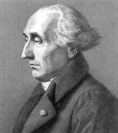It’s useful to have the theory described in terms of a scalar quantity, because it doesn’t matter what coordinate system you view it in. To see this, imagine standing on your head while I throw a ball up in the air. To me, it appears that the ball moves upwards, while to you, it appears it moves downwards. However, if we choose a scalar quantity such as its mass, we will both agree on this.

The force on an object and its resulting acceleration are vectors associated with a particular point – the point at which that object is located. Similarly, its mass is a scalar associated with a particular point.

## Fields

However, it’s also possible to define scalar and vector “fields”, which take values at every point across a space. You can see a scalar field and a vector field every day on the weather forecast. The map showing temperatures across the country shows a scalar field, while the map showing wind velocities across the country shows a vector field.Temperature as a function of position – a scalar field [Attribution]

Gravity can also be described – somewhat helpfully – as a field. Any physical object is viewed as surrounded by a gravitational field. For example, consider a ball that has been thrown by someone standing on the Earth’s surface. The Earth and the ball both have gravitational fields. But Newtonian theory says that the higher the object’s mass, the stronger the field. This means that the gravitational field of the Earth is far stronger than that of the ball. Thus the force pulling the ball towards the Earth is much greater than the force pulling the Earth towards the ball. The field also drops off with distance from the object – this is why on a trip to the moon, astronauts experience “weightlessness” when the rocket’s engines aren’t firing.

The way the gravitational field depends on the distribution of mass generating it is described by a “field equation” called Poisson’s equation.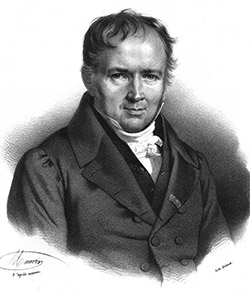## Other fundamental interactions

Besides gravity, only one other fundamental interaction was known about at the end of the 19th Century. This was electromagnetism.

An object which carries an electromagnetic charge is surrounded by an electric field. This is stronger when the charge is greater and, like the gravitational field, drops off with distance. Similarly, a magnet is surrounded by a magnetic field, which is stronger for more powerful magnets and drops off with distance.

However, these are not entirely separate fields. For example, in a dynamo, magnets are used to generate an electric current.The relations between electric and magnetic phenomena were figured out over time by a number of physicists. This culminated in the idea of a single electromagnetic field, again described in field equations, proposed by James Clerk Maxwell.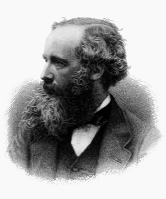In the early decades of the 20th Century, physicists discovered the structure of the atom – that it’s composed of protons, neutrons and electrons – and how this structure can change through radioactivity. They realised that two more interactions were involved. The “strong interaction” binds the atomic nucleus together, while the “weak interaction” is responsible for a particular type of radioactive decay, known as “beta decay”.

Furthermore, the classical mechanics of Newton, even using Lagrange’s recasting of it, was insufficient for describing the structure of the atom and any mechanics happening at these small scales. Instead, a new type of mechanics, quantum mechanics, was needed.

There are many excellent books on quantum mechanics, both for the lay reader and for physicists, and I won’t cover it in these pages. For the lay reader, I’d recommend The Quantum Universe by Tony Hey and Patrick Walters – a great coffee table book which explores all the oddities of the quantum realm. My undergraduate course text was Quantum Mechanics by Sara M. McMurry, which seemed to cover all I needed during my undergraduate years.

## Special relativity

Meanwhile, Albert Einstein was exploring what happens at high speeds and in strong gravitational fields.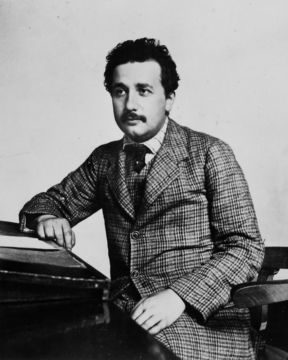He had noticed something strange about Maxwell’s electromagnetic theory. It was well known that “ripples” could propagate through the electromagnetic field, or electromagnetic waves as they’re usually known. (These waves, depending on their frequency, are radio waves, infrared, visible light, ultraviolet, X-rays or gamma rays.) The equation of motion for these waves could be derived from Maxwell’s field equations. One feature of it is that the waves always move at a fixed speed, known as the speed of light. (More precisely, they move at a fixed speed in a particular medium. They move at their fastest speed in empty space but are slightly slowed down when moving through matter such as air or water.) This speed is the same for all observers.

To see how odd this is, consider an open-top car going along a straight road at 50mph. Next to the driver, there is an extremely foolish passenger, standing up in his seat and behaving recklessly. The passenger throws an object out of the car.

First, let’s say he throws it forward, in the direction the car is travelling, at 30mph. This object moves away from the passenger at a speed of 30mph with respect to that passenger. The speed it travels with respect to the road is simply the sum of these: 50mph + 30mph = 80mph. If, for example, there is a person standing at the side of the road with a speed gun, they would measure the velocity of the object as 80mph (assuming the speed gun to be accurate!).

Now let’s say the passenger throws the object back along the road, in the direction that the car has come from, at 30mph. Now it moves at 30mph with respect to the passenger, but at 50mph – 30mph = 20mph with respect to the road.

However, we now consider the situation where the passenger has a torch. He shines the torch at the road ahead of him. The light leaving the torch leaves the torch at the speed of light (in air) with respect to the passenger, but according to Maxwell, it also moves at the speed of light with respect to the road. Similarly, if he shines it behind the car, it moves at the speed of light with respect to both the passenger and the road. The addition of velocities simply doesn’t work for light.Two observers in relative motion observe light as moving at the same velocity (in this case, in rockets, measuring the velocity of light c leaving the sun) [Attribution]

Having spotted this, Einstein thought about what it would be like to travel around on a particle of light – a photon. These thoughts led to the Special Theory of Relativity (often simply known as “special relativity”). This contains many other counter-intuitive features, relating to measurements of quantities such as length, time, momentum and kinetic energy by observers in relative motion. If these observers are travelling at a constant velocity with respect to each other, and this velocity is a significant fraction of the velocity of light, these measurements will differ significantly. I won’t go through the details of this here, but again there are excellent books on the subject – for the lay reader, I’d highly recommend Relativity for the Layman by James A. Coleman.

The theory was set out in two papers published in 1905. In this theory, measurements were made in the usual three dimensions of space. These position or length measurements were supplemented by recording the time on (moving) clocks. However, in 1907 Hermann Minkowski pointed out that the equations in the papers could be derived very simply if one considered time and three-dimensional space as parts of a larger four-dimensional “spacetime”.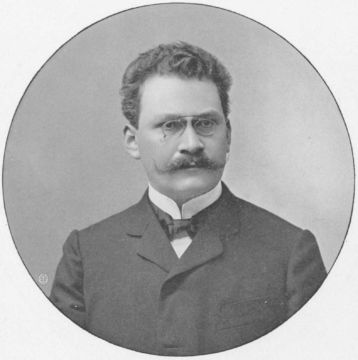A key concept here is that of “frames of reference”. Going back to the image of me throwing up a ball while you’re standing on your head, your “frame of reference” – the way you see the world – is rotated with respect to mine. (In simple language, it is turned upside-down.) This rotation of the reference frame is the reason that the ball appears to move up in my reference frame and down in yours. That is, our measurements of the ball’s velocity are “equal and opposite”. Similarly, the measured velocity of the object the passenger threw from the car depends on your frame of reference – the passenger’s frame of reference is moving at a constant velocity with respect to that of the person standing at the side of the road. In Minkowski’s spacetime mathematics, the change between these two reference frames is very similar to a rotation. Indeed, this change could reasonably be described as a “rotation in spacetime”.

## General relativity

Special relativity only described measurements by observers moving at a constant velocity with respect to each other. Einstein wanted to include measurements by observers whose velocity varied – in other words, accelerating or decelerating with respect to each other. This necessarily meant considering forces. If you’re in an aeroplane that is launching rapidly, you will feel forced back into your seat. This force could be measured, just as weight can be measured.

Here, again, Einstein spotted something curious. If you were in a lift and somebody cut the cable, as the lift fell, everything in it would become “weightless”. Things would float around in front of you, just as if somebody had switched gravity off. Conversely, if you were in a spaceship in deep space, you would float around weightless, until the spaceship started accelerating. If it accelerated at the right rate in the right direction, you’d feel as if gravity had been restored. You could walk around on the floor just as if you were standing on the Earth. Furthermore, anyone and anything in the spaceship would experience the same – no matter whether they were a flea or an elephant.

The reason for this is that the force exerted by gravity on an object is proportional to its mass. Then, as the acceleration caused by a force is equal to that force divided by the object’s mass, every object accelerates at the same rate. If you were to suck all the air out of a lift shaft running down a skyscraper and drop a hammer and a feather from the top, they would take the same length of time to reach the bottom. This feature isn’t true of other forces. For example, the electrostatic force acting on an object is proportional to its electric charge, not its mass.

Einstein therefore came to a radical conclusion. He was looking for a theory which related measurements by observers accelerating with respect to each other. He concluded that this would need to describe gravity.

It took him until 1915 to develop the theory. The General Theory of Relativity (or “general relativity”) proposed that massive objects cause spacetime to curve or warp. This is often described using the “rubber sheet analogy”. If you take a rubber sheet, or something similar like a trampoline, and place a cannonball on it, you’ll warp its formerly flat surface. Any other object in the neighbourhood will no longer move in a perfectly straight line. If you were to roll a marble across the sheet, for example, it would follow a curve around the warped part of this sheet. In general relativity, this is what happens in the presence of a massive object.

For example, in our solar system, planets and comets follow curves around the sun. Even light bends round stars:

Similarly, if I stand on the surface of the Earth and throw a ball, the Earth’s high mass causes a curvature of the surrounding spacetime and this results in the ball following a curved path.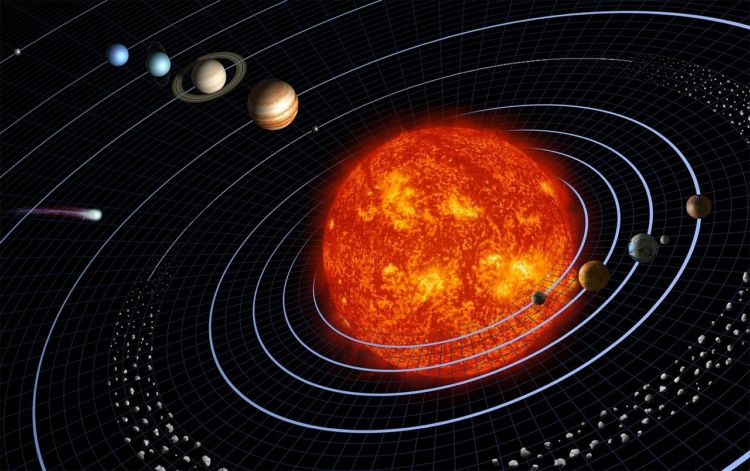The pinnacle of the theory were its field equations, which described the relation between a matter distribution and the curvature it causes. As the physicist John Archibald Wheeler succinctly summed it up, “Spacetime tells matter how to move; matter tells spacetime how to curve”.

## Tangent spaces

Curved spaces, such as the surface of the earth or spacetime in general relativity, are generally difficult to analyse. Analysis is generally performed by considering tangent spaces and the relations between them. In the case of the Earth’s surface, the tangent space at a given point is an imaginary two-dimensional flat plane which touches the surface only at that point. At a small enough scale, this plane will be indistinguishable from the surface itself. Thus a map of your locality is essentially an example of a tangent space.

One can define “tangent vectors” in a tangent space – that is, vectors which lie in that tangent space. A vector field then has a specific value in each tangent space – that is, in the space tangent to each point on the underlying curved space.

The study of such features of curved spaces is an area of mathematics known as differential geometry. General relativity employs various quantities which were already in use in differential geometry. One of these, the metric, is used in calculating the length of a vector in any given tangent space. To compare vectors in different tangent spaces, you need another quantity called a connection. It is actually possible to define many different types of connection; general relativity uses one called the Levi-Civita connection.

## Extending General Relativity

After publishing his General Theory of Relativity in 1915, Einstein spent much of the next 15 years investigating certain ideas as to how the theory could be extended to incorporate electromagnetism. The greatest advances in this at this time were made by Theodor Kaluza and Oscar Klein. The theory they developed applied general relativity to a five-dimensional space-time. The extra dimension had a condition applied to it that if you went an absolutely tiny distance along it, you ended up back where you started. By “absolutely tiny”, I mean the following. When Klein calculated this distance, he found it to be smaller than the diameter of the nucleus of an atom, by roughly the same factor that the diameter of the nucleus of an atom is smaller than the height of you or me.

A different approach Einstein tried was to use a different type of connection; this is now generally known as the Weitzenböck connection. This approach has been expanded by other researchers over the years to create a new branch of physics known as teleparallelism. They have developed the Teleparallel Equivalent of General Relativity (TEGR), which uses teleparallelism to derive field equations which are equivalent to those of general relativity.

Further information on these connections can be found in the following preprint:

Preprint of an article published in International Journal of Geometric Methods in Modern Physics, 2021 https://doi.org/10.1142/S0219887821400089 © World Scientific Publishing Company

You can continue reading in my Breaking page: